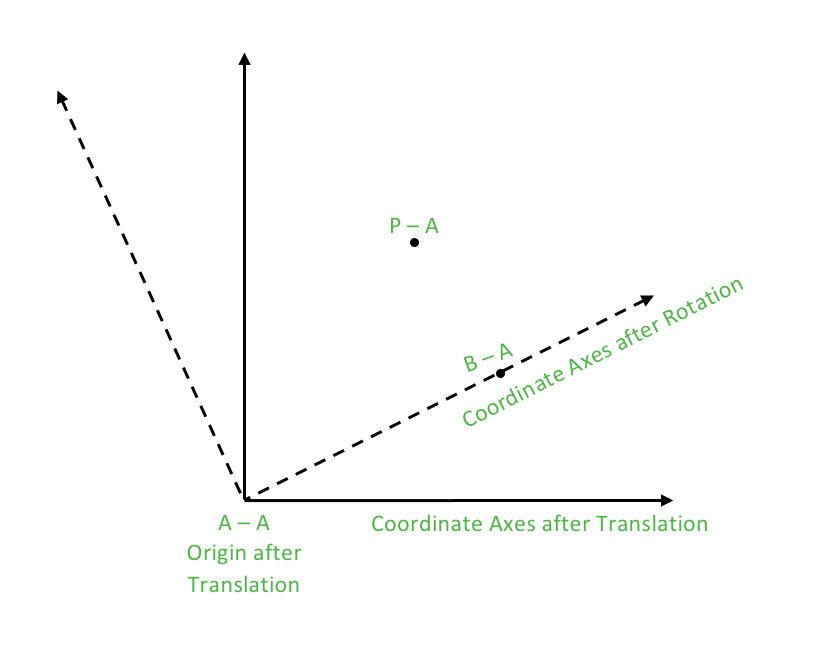GeeksforGeeks App
Open AppBrowser
Continue

# Reflection of a point about a line in C++

Let’s first consider a general case where the line is nothing but the X-Axis. We can now definitely say that the conjugate of a point is the reflection of the point about X-Axis.
Now, using the methods of translation and rotation of coordinate axes we will find out the reflection of a point about the generic line.
The idea of translation was described in the previous post. Here we describe the idea of rotation.
What is Rotation?
In Euclidean geometry, a rotation of axes in two dimensions is a mapping from an xy-Cartesian coordinate system to an x’y’-Cartesian coordinate system in which the origin is kept fixed and the x’ and y’ axes are obtained by rotating the x and y axes through an angle θ.
How to Perform Rotation?
Rotation can be interpreted as multiplying (rotating in anticlockwise direction) or dividing (rotating in clockwise direction) every point of the coordinate system by a constant vector.
Note here that if we want to rotate a point by θ in the anticlockwise direction about the origin, we multiply it by polar (1.0, θ) as discussed in SET 1. Similarly, we divide by polar (1.0, θ) to rotate the point by θ in the clockwise direction.
After the rotation, required computations are performed and rotation is nullified by dividing or multiplying every point by the constant vector respectively.
So, we have to reflect a point P about a line specified by points A and B denoted as AB. Since, we know that the conjugate of a point is the reflection of the point about X-Axis. In order to be able to use this fact, we will first perform translation (making A as the origin in the new system) and then rotating the coordinate axes in such a way that the line becomes the X-Axis in the new coordinate system.
Now we can simply apply the formula for reflection about X-Axis and then nullify the effects of rotation and translation to get the final result.
These steps can be described as under:

1.Translation (Shifting origin at A): Subtract A from all points.

```Pt = P – A
Bt = B – A
At is origin```

2.Rotation (Shifting BtAt to the X-Axis): Divide all points by Bt (dividing means rotating in clockwise direction which is the requirement here to bring on X-Axis).

`Pr = Pt/Bt `

3.Reflection of Pr about BrAr (which is nothing but the X-Axis): Simply take the conjugate of the point.

`Prreflected = conj(Pr) `

4.Restoring back from Rotation: Multiply all points by Bt.

`Ptreflected= conj(Pr)*Bt `

5.Restoring back from Translation: Add A to all points.
P reflected = conj(Pr)*Bt + A

Thus,

```return conj(Pr)*Bt + A
where, Bt = B – A
Pt = P – A
Pr = Pt/Bt```## CPP

 `// CPP example to illustrate the``// reflection of a point about a line``#include ``#include ` `using` `namespace` `std;` `typedef` `complex<``double``> point;``#define x real()``#define y imag()` `// Constant PI for providing angles in radians``#define PI 3.1415926535897932384626` `// Function used to display X and Y coordinates of a point``void` `displayPoint(point P)``{``    ``cout << ``"("` `<< P.x << ``", "` `<< P.y << ``")"` `<< endl;``}` `// Function for Reflection of P about line AB``point reflect(point P, point A, point B)``{``    ``// Performing translation and shifting origin at A``    ``point Pt = P-A;``    ``point Bt = B-A;` `    ``// Performing rotation in clockwise direction``    ``// BtAt becomes the X-Axis in the new coordinate system``    ``point Pr = Pt/Bt;` `    ``// Reflection of Pr about the new X-Axis``    ``// Followed by restoring from rotation``    ``// Followed by restoring from translation` `    ``return` `conj(Pr)*Bt + A;``}` `int` `main()``{``    ``// Rotate P about line AB``    ``point P(4.0, 7.0);``    ``point A(1.0, 1.0);``    ``point B(3.0, 3.0);` `    ` `    ``point P_reflected = reflect(P, A, B);``    ``cout << ``"The point P on reflecting about AB becomes:"``;``    ``cout << ``"P_reflected"``; displayPoint(P_reflected);` `    ``return` `0;``}`

Output:

`The point P on reflecting about AB becomes: P_reflected(7, 4)`

Time Complexity: O(1)
Auxiliary Space: O(1)

This article is contributed by Aarti_Rathi and Aanya Jindal. If you like GeeksforGeeks and would like to contribute, you can also write an article using write.geeksforgeeks.org or mail your article to review-team@geeksforgeeks.org. See your article appearing on the GeeksforGeeks main page and help other Geeks.